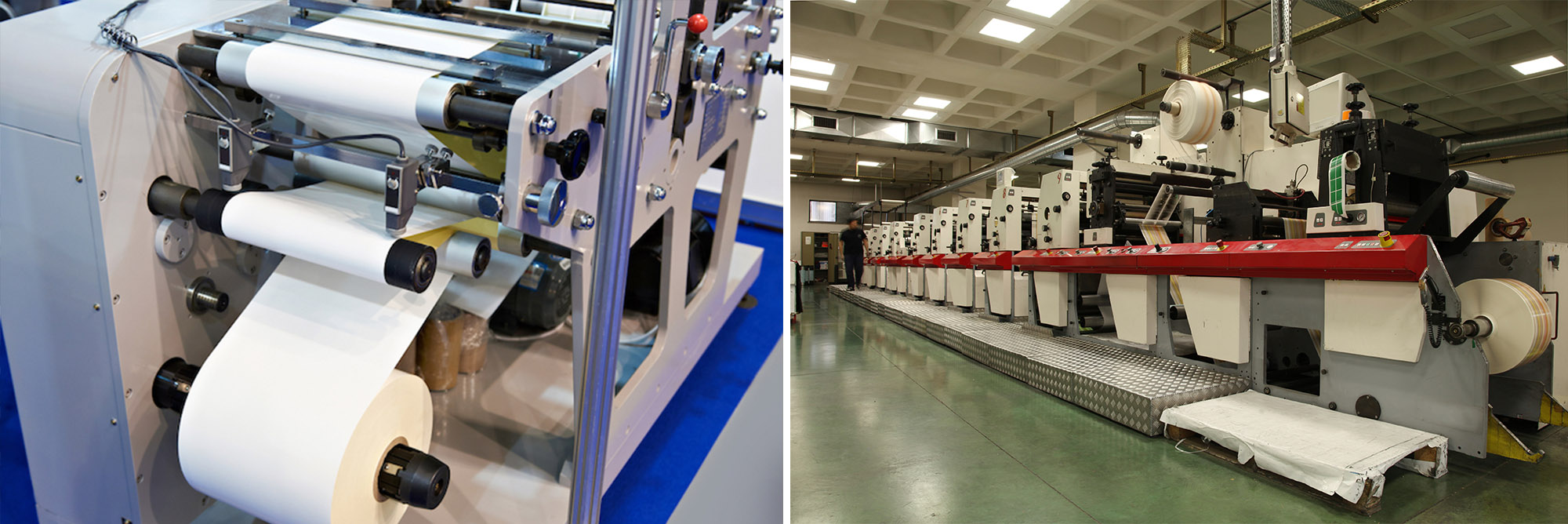# Selecting the Right Clutches and Brakes for Web Tension Control — Part IIIn our last blog post, we explored how to select your clutch to maintain and control web tension in printing, coating, laminating and converting processes. Now, let’s turn to brakes.

As the material being processed in these applications depletes from the input roll, causing the roll diameter to shrink, the torque required to maintain tension on the roll decreases in a linear ratio. Your brake’s torque output must decrease accordingly whether manually or automatically. To determine the required torque range, simply perform the following calculations:

1. Determine the required tension. Tension is measured in pounds per linear inch (PLI).

If the PLI is unknown, then:
PLI = Total Tension (pounds) / Web Width (inches)
For example: 0.5 PLI = 6.5 lbs / 13 in

If the PLI is known, then:
Total Tension (pounds) = PLI x Web Width (inches)
For example: 6.5 lbs = 0.5 PLI x 13 in

2. Next, calculate the required torque range.

The Maximum Required Torque (lb-in) = Total Tension (pounds) x Radius at Full Roll (inches)
For example: 156 lb-in = 6.5 lbs x 24 in

The Minimum Required Torque (lb-in) = Total Tension (pounds) x Radius at Core (inches)
For example: 19.5 lb-in = 6.5 lbs x 3 in

Unless your machine is dedicated to only one product, then you must also consider the PLI range, web widths, core and maximum roll diameters. Determine the required torque range for each product that will be processed on the machine.

A brake used on the unwind shaft is typically driven by the machine’s primary drive at a constant input speed, which is expressed in rotations per minute (RPM). As the material feeds into the machine and the roll diameter decreases, the roll RPM increases. Your brake must compensate for the difference between the input and output RPM by slipping. For the unwind section, this process is happening as the required torque decreases. As a result, you only need one calculation to determine the thermal load.

Thermal load is expressed in thermal horsepower (Thp), but it can also be expressed in slip watts or kilowatts depending on the manufacturer:

Heat (Thp) = Total Tension (pounds) x Linear Speed (feet per minute) / 33,000

For example: 0.05 Thp = 6.5 lbs x 250 fpm / 33,000

Be sure to refer to your manufacturer’s thermal ratings to determine if your brake has the heat capacity for the intended process. Unless your machine is dedicated to only one product, then you must also consider the PLI range, web widths, core and maximum roll diameters. Determine the required heat dissipation for each product that will be processed on the machine.